Next: The Motivation for an Alternative Pairing Mechanism Up: The Pairing Mechanism Previous: The Pairing Mechanism

## s-Wave Pairing

As touched upon earlier, conventional BCS superconductors are characterized by a standard s-wave, spin-singlet pairing state with S=0, L=0 Cooper pairs. The two electrons of a pair have equal and opposite momenta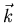and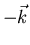, so that the centre-of-mass momentum of a Cooper pair is zero. For an attractive electron-electron interaction, the bound state is symmetric upon exchange of electron positions, so it must be an antisymmetric singlet upon exchange of electron spins to satisfy the Pauli exclusion principle. Thus, at any instant of time, one can think of the electrons in a Cooper pair as being in a state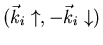, and the wavefunction describing the pair consists of all states i'' occupied by the pair during its lifetime. An attractive interaction between two electrons results in a potential energy contribution which is negative, and thus lowers the total energy of the electron system. The negative potential energy associated with a Cooper pair is the binding energy of that pair.

In normal metallic superconductors, the attractive interaction between electrons originates from the electron-phonon interaction, which is short range and retarded in space-time . The lattice deformation resulting from the first electron takes a finite time to relax, and thus a second electron can be influenced by the lattice deformation at a later time. The attraction arising from the electron-phonon interaction overcomes the screened Coulomb repulsion between electrons due to the presence of other electrons and ions in the solid . This screening aids in reducing the natural Coulomb repulsion between two electrons, leading to an effective interaction which is relatively short range compared with the unscreened Coulomb potential .

The net effect of the attractive interaction on all the other electrons in the material renders the normal Fermi liquid state unstable. Consider the normal ground state of a metal in the absence of an attractive electron-electron interaction at T=0K. The kinetic energy (and hence the total energy) of the system is minimized by requiring that the momenta of the plane wave states of the electrons fill up a sphere of radius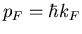(i.e. a Fermi sphere) in three-dimensional momentum space. Fig. 2.6(a) illustrates a Fermi sphere of radius kF in three-dimensional k-space for a free electron gas at T=0K. The corresponding normal density of states N(E), where N(E)dE is defined as the number of electron states with energy between E and E+dE, is shown in Fig. 2.6(b). N(0) denotes the density of states at the Fermi surface at absolute zero.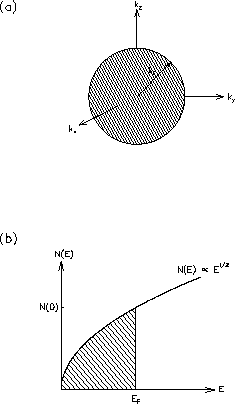Original BCS theory described superconductivity in metals, so that the Fermi surface was assumed spherical in the normal state. In the BCS ground state (i.e. the state of the superconductor at T=0K), some of the electron states just outside the normal Fermi surface are occupied, and some just inside the Fermi surface are unoccupied. Certainly such an arrangement has a higher kinetic energy than does the normal state of the metal at T=0K. However, the BCS ground state is in part comprised of Cooper pairs, the formation of which lowers the potential energy of the system. In the BCS ground state the arrangement of Cooper pairs is such that lowering of the potential energy outweighs the increase in kinetic energy, so that the BCS ground state has a lower total energy than that of the normal ground state .

As mentioned, the decrease in potential energy is due to the phonon-induced, electron-electron attractive interaction. The phonon-induced attractive force scatters Cooper pairs from one state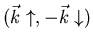to another state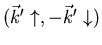. An electron occupying state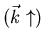near the Fermi surface vibrates the lattice resulting in the emission of a phonon of wave vector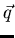. The electron is thereby scattered to a state, where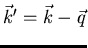. A second electron occupying state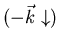, absorbs the phonon thus scattering to a state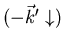, where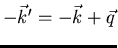. The total centre-of-mass momentum in the final state is thus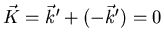, which is unaltered from before the scattering process. The two electrons forming a Cooper pair are continually scattered between states with equal and opposite momentum. Since only the total centre-of-mass momentum is conserved in such scattering processes, the momenta of the individual electrons is continually changing, so that one cannot explicitly assign a single momentum to each electron in the pair.

Each scattering process reduces the potential energy of the electron system further. The negative potential energy contribution is greatest for scattering between states of equal and opposite momentum . The range of momenta available to the scattered electrons in the ground state is dictated by the energy of the phonon and the Pauli exclusion principle. The phonon-induced attractive interaction can only affect those electrons in the vicinity of the Fermi surface. Electrons further inside the Fermi sphere cannot scatter to other levels because of the Pauli exclusion principle. To scatter electrons well below the Fermi level into unoccupied states would require phonon frequencies much larger than that generated from the electron interaction with the lattice. Electrons closer to the Fermi surface may form Cooper pairs; however, as they do so the number of states available to the scattering electrons decreases. As the number of scattering events decreases, so does the maximum amount by which the potential energy of the system can be lowered. The formation of Cooper pairs must then cease at the moment the increase in kinetic energy due to moving electrons above the Fermi level exceeds the amount by which the potential energy is lowered. This limit then specifies the arrangement of the BCS ground state. One of the most distinctive consequences of BCS theory is that an energy gap opens up between the ground state and the lowest excited state. As shown in Fig. 2.7(a), the energy gap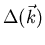has the same symmetry as the Fermi surface of the normal state. The important feature in s-wave pairing is that the wave vector dependence of the energy gap is finite everywhere on the Fermi surface at temperatures below Tc.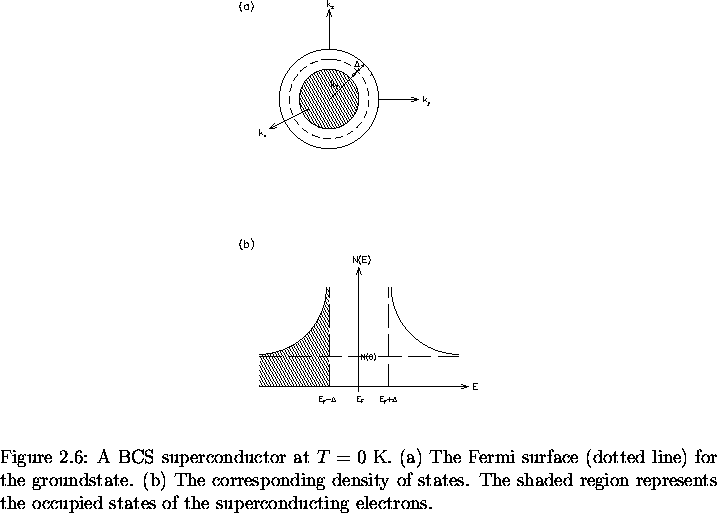As the temperature is increased above T=0K, thermally excited phonons become available to scatter the electron pairs. Because of the energy gap in the excitation spectrum, excitations cannot occur with an arbitrarily small amount of energy as in the case of a normal metal. Phonons with energy comparable to twice the energy gap (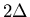) will scatter electrons in a Cooper pair to states above the gap. The electrons from the pair will no longer have equal and opposite momentum, so that their interaction potential becomes negligible and the Cooper pair is destroyed. Of course at low temperatures, the density of phonons with this much energy is small. Near Tcon the other hand, phonons with energy on the order of the energy gap are plentiful, and pair breaking is greatly enhanced. In addition, the gap itself is temperature dependent and can be well approximated by :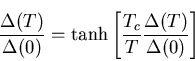(30)

except near Tc where Eq. (2.18) better describes the behaviour. As T increases, the amplitude and frequency of the lattice-ion movement increases. This disrupts the propagation of the phonons between correlated electron pairs, resulting in a weakening of the attractive interaction and a corresponding reduction in the size of the energy gap .

Fig. 2.7(b) and Fig. 2.8 show the associated density of quasiparticle states N(E)at T=0K and T slightly above absolute zero, respectively. The states which are no longer occupied between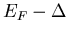and EF+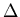pile up on the edges of the gap region. Above the energy gap, the electrons are often referred to as normal electrons or quasiparticles. That is, if an electron occupies a statethen the stateneed not be occupied. The corresponding density of quasiparticles (i.e. the normal fluid density) is usually denoted nn. Below the energy gap the electrons are paired. These electrons are referred to as superconducting electrons, and their associated density (i.e. the superfluid density) is denoted ns. At T=0K all the electrons are superconducting, while for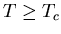they are all normal. For temperatures in between, the system is a mixture of superconducting and normal electrons.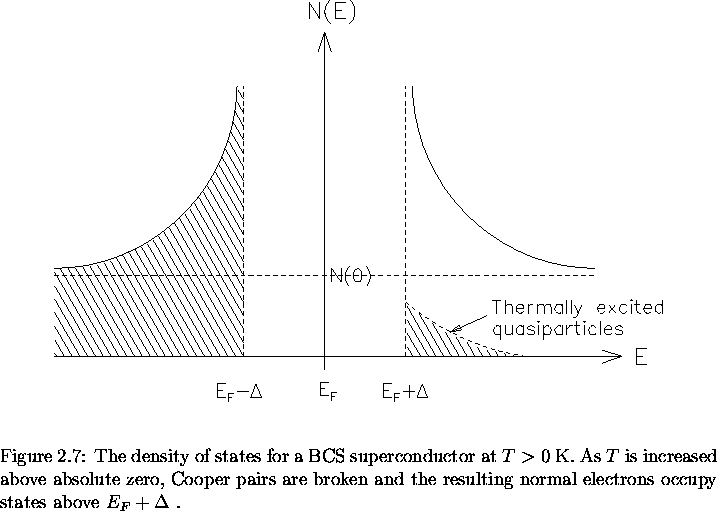Next: The Motivation for an Alternative Pairing Mechanism Up: The Pairing Mechanism Previous: The Pairing Mechanism
Jess H. Brewer
2001-09-28